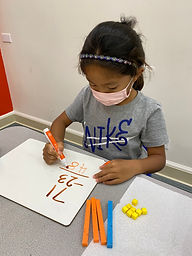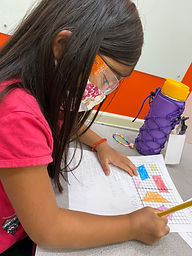Ms. Brianne

Target 1​

Lesson Type:

New

Number Operation

:

Computation

Subtract within 1,000 (regrouping limited to only one place-value).

1:

Understand why the standard algorithm for subtraction is based on place-value.

2:

Understand that when subtracting a multi-digit number, each place-value is being subtracted individually to create the new number (ones are subtracted from ones, tens are subtracted from tens, etc.)

3:

Understand that when adding a multi-digit number, to solve start in the lowest place-value and go left.

4:

Understand how to regroup based on place-value understandings (i.e., ten ones can be composed into one ten – ten tens can be composed into one hundred).

2nd

Vocabulary:

Subtract, Regroup, Ones, Tens

Activities:

• Students solved two-digit subtraction problems involving regrouping.
• Students used place value and base ten blocks to build the numbers and act out subtraction.
• Students used regrouping when necessary, trading 1 ten for 10 onesHome Exploration

Guiding Questions:Absent Students:

Target 2

:

1:

Understand that geometric figures can be drawn on the coordinate plane.

2:

Become familiar with how the coordinate plane can be used to determine distance and length of geometric shapes.

2nd

Vocabulary:

Coordinates, Coordinate pairs, Coordinate plane, X-axis, Y-axis, 2D shapes

Activities:

• Students named the coordinate pairs for different locations on the coordinate grid.
• Students were given sets of coordinates to plot and connect on a coordinate plane to figure out the mystery shapes.Home Exploration

Guiding Questions:Target 3

:

Vocabulary:

Activities:Home Exploration